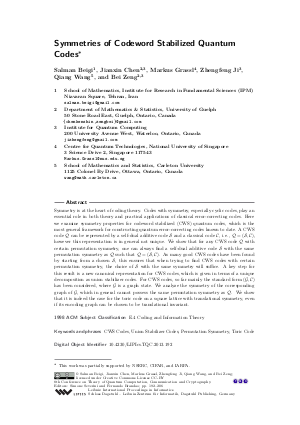Document# Symmetries of Codeword Stabilized Quantum Codes

### Authors Salman Beigi, Jianxin Chen, Markus Grassl, Zhengfeng Ji, Qiang Wang, Bei Zeng## File

LIPIcs.TQC.2013.192.pdf
• Filesize: 0.6 MB
• 15 pages

## Cite As

Salman Beigi, Jianxin Chen, Markus Grassl, Zhengfeng Ji, Qiang Wang, and Bei Zeng. Symmetries of Codeword Stabilized Quantum Codes. In 8th Conference on the Theory of Quantum Computation, Communication and Cryptography (TQC 2013). Leibniz International Proceedings in Informatics (LIPIcs), Volume 22, pp. 192-206, Schloss Dagstuhl - Leibniz-Zentrum für Informatik (2013)
https://doi.org/10.4230/LIPIcs.TQC.2013.192

## Abstract

Symmetry is at the heart of coding theory. Codes with symmetry, especially cyclic codes, play an essential role in both theory and practical applications of classical error-correcting codes. Here we examine symmetry properties for codeword stabilized (CWS) quantum codes, which is the most general framework for constructing quantum error-correcting codes known to date. A CWS code Q can be represented by a self-dual additive code S and a classical code C, i.e., Q=(S,C), however this representation is in general not unique. We show that for any CWS code Q with certain permutation symmetry, one can always find a self-dual additive code S with the same permutation symmetry as Q such that Q=(S,C). As many good CWS codes have been found by starting from a chosen S, this ensures that when trying to find CWS codes with certain permutation symmetry, the choice of S with the same symmetry will suffice. A key step for this result is a new canonical representation for CWS codes, which is given in terms of a unique decomposition as union stabilizer codes. For CWS codes, so far mainly the standard form (G,C) has been considered, where G is a graph state. We analyze the symmetry of the corresponding graph of G, which in general cannot possess the same permutation symmetry as Q. We show that it is indeed the case for the toric code on a square lattice with translational symmetry, even if its encoding graph can be chosen to be translational invariant.
##### Keywords
• CWS Codes
• Union Stabilizer Codes
• Permutation Symmetry
• Toric Code

## Metrics

• Access Statistics
• Total Accesses (updated on a weekly basis)
0